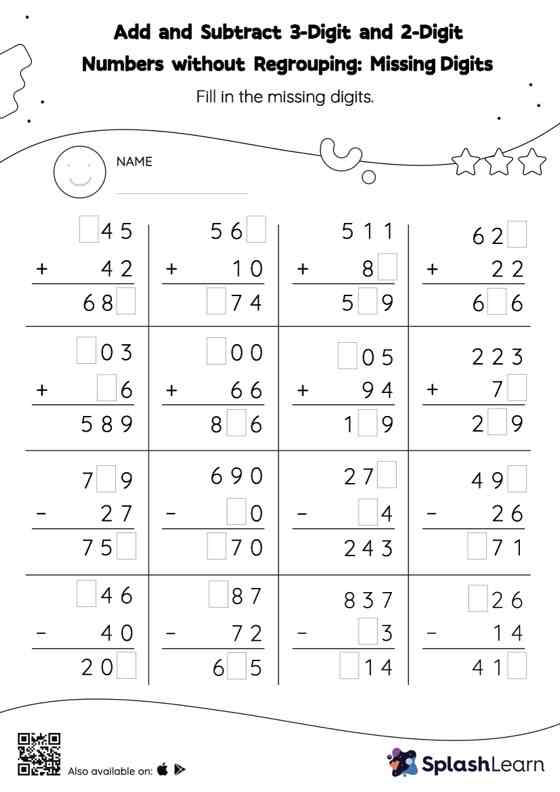# Add and Subtract 3-Digit and 2-Digit Numbers without Regrouping: Missing Digits Worksheet

Home > Add and Subtract 3-Digit and 2-Digit Numbers without Regrouping: Missing DigitsIn this worksheet, students must find the missing number using the relationship between addition and subtraction. To solve add and subtract 3-digit and 2-digit numbers without regrouping worksheet, they do not need to regroup the numbers. As the worksheet uses the column method, it is helpful in getting students toward higher accuracy, especially with bigger numbers and in scenarios where regrouping is required.